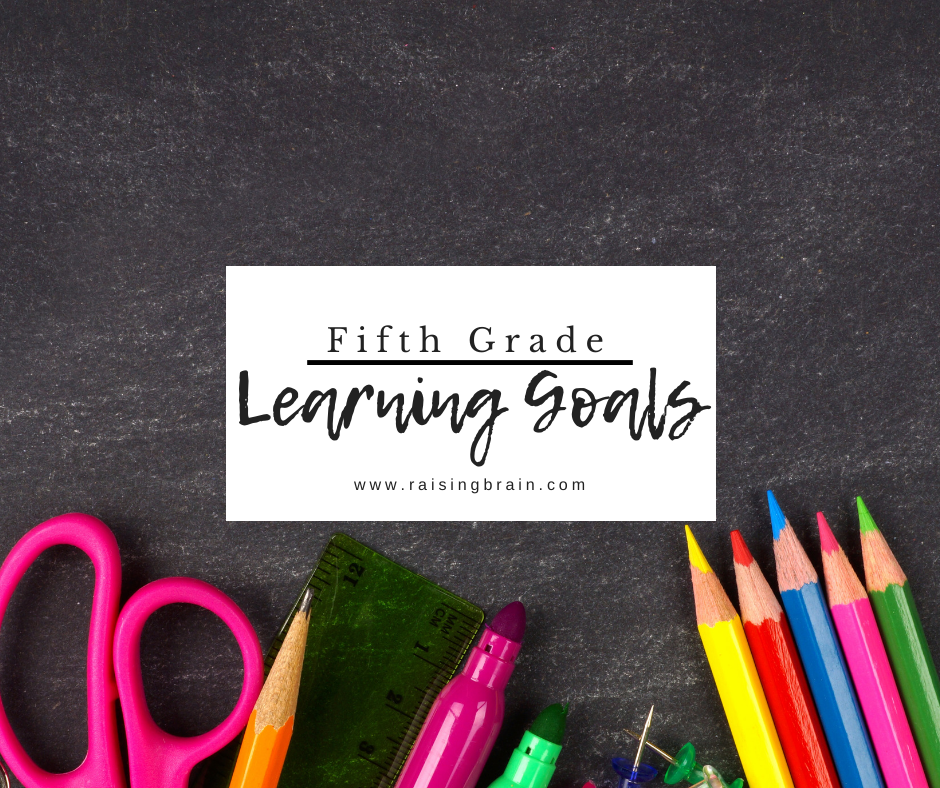In fifth grade, students will practice more complex mathematical concepts such as exponents, decimals, fractions and more. They will read and understand new vocabulary in science and social studies as well significantly improve their grammar skills. They will expand their knowledge about the scientific method, historical events and important historical figures.

Below are some of the skills and concepts a fifth grade education should include.

• Comparing and contrasting topics and themes

• Using linking words and writing a conclusion

• Multiplying two fractions using an area model

• Understanding how Earth’s four spheres interact within the water cycle

• Knowing and locating different climate zones around the world

## Math Objectives for 5th Grade

Below are just some of the 5th grade math objectives your child is expected to achieve. Finding a comprehensive and effective fifth grade math curriculum will ensure your child is learning all the important concepts and topics necessary to move on to sixth grade.

• Use place value to add, subtract, multiply and divide decimals

• Apply formulas to solve various customary length/weight/capacity/temperature problems

• Calculate the probability of an event

Below are some concepts a fifth grader should know.

• Rounding numbers to the nearest ten, hundred, thousand, ten thousand and hundred thousand

• Using partial products and mental multiplication

• Dividing with two-digit divisors

• Ordering and comparing fractions

• Comparing perimeter and area